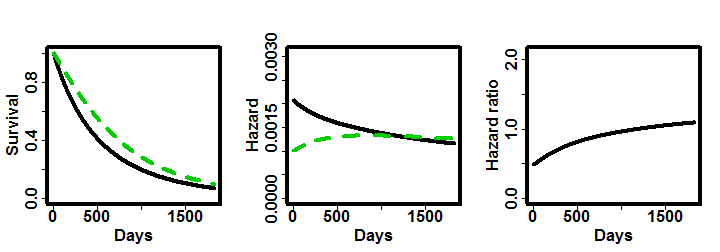In this vignette we showcase the various options to specify non-proportional hazards with the nph package.

library(nph)

Consider a clinical trial in which patients are randomised to either an experimental treatment or a control one. The considered endpoint is survival time and the follow-up time is 5-years.

Progression of disease

The first example we consider is non-proportional hazards due to the case when there is progression of disease that affects the survival of the patients. Moreover, since the progression appears with different rates for treatment and control arms, it leads to a violation of the non-proportional assumption.

As an example, consider the case where the median time to progression of disease is 9 montths for the experimental treatment (TRT) and 5 months for the control one (CTRL). Before progression, the median overall survival (OS) times are 18 (TRT) and 9 (CTRL) and after progression they decrease to 11 (TRT) and 9 (CTRL).

This is example is roughly based on the results observed in the study Keynote 189 (NEJM issue:22; 2018)

times <- c(0, 5 * 365)   # Time interval boundaries, in days

# Treatment group
t_resp <- 1              # There are no subgroups
B5 <- pop_pchaz(
T = times,
lambdaMat1    = m2r(matrix(18,nrow = 1)),
lambdaMat2    = m2r(matrix(11,nrow = 1)),
lambdaProgMat = m2r(matrix(9, nrow = 1)),
p = t_resp,
timezero = FALSE, discrete_approximation = TRUE
)

# Control group
c_resp <- 1              # There are no subgroups
K5  <- pop_pchaz(
T = times,
lambdaMat1    = m2r(matrix(11,nrow = 1)),
lambdaMat2    = m2r(matrix(9, nrow = 1)),
lambdaProgMat = m2r(matrix(5, nrow = 1)),
p = c_resp,
timezero = TRUE, discrete_approximation = TRUE
)

pp = plot_diagram(B5, K5)
pp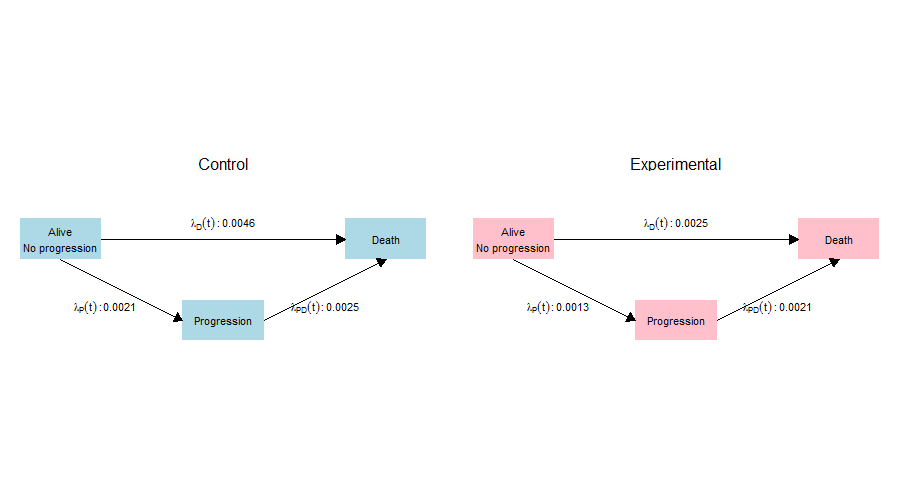The plot shows us the the survival and hazard functions by group, together with the hazard ratio. We observe that the HR is not constant through time and conclude that progression of disease leads to a non-proportional hazard.

plot_shhr(K5, B5)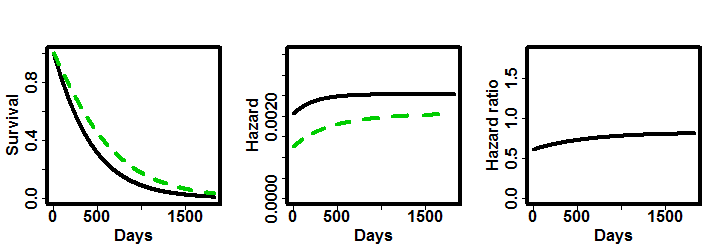Subgroup with differential effect

Another feature that may lead a violation of the non-proportional hazards assumption is the presence of predictive subgroup. That is, subgroups with a differential treatment effect. We then build on the previous example to include a subgroup (with prevalence of 20%) with an additional benefit. The median time to progression in the subgroup is 15mo, while the median OS is 30mo before progression and 20mo after progression. For the complement of the subgroup, the parameters are maintained at the values of the previous example.

times <- c(0, 5 * 365)   # Time interval boundaries, in days

# Treatment group
t_resp <-  c(.2, .8)
B5 <- pop_pchaz(
T = times,
lambdaMat1    = m2r(matrix(c(30,
18),nrow = 2)),
lambdaMat2    = m2r(matrix(c(20,
11),nrow = 2)),
lambdaProgMat = m2r(matrix(c(15,
9), nrow = 2)),
p = t_resp,
timezero = FALSE, discrete_approximation = TRUE
)

# Control group
c_resp <- 1
K5  <- pop_pchaz(
T = times,
lambdaMat1    = m2r(matrix(11,nrow = 1)),
lambdaMat2    = m2r(matrix(9, nrow = 1)),
lambdaProgMat = m2r(matrix(5, nrow = 1)),
p = c_resp,
timezero = TRUE, discrete_approximation = TRUE
)

pp = plot_diagram(B5, K5, A_subgr_labels = c("S1", "S2"))
pp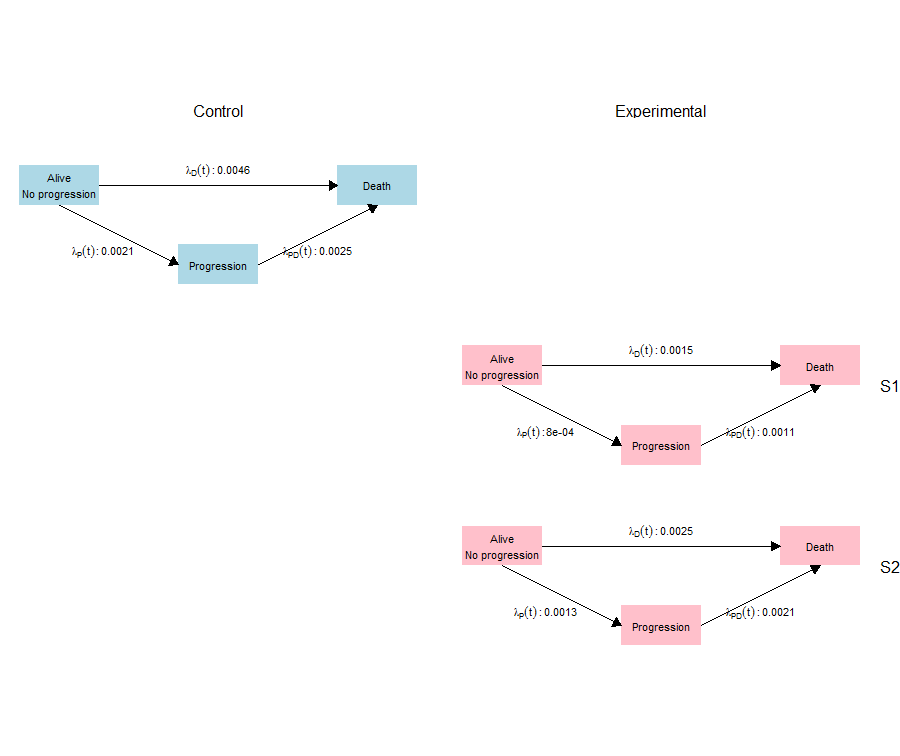plot_shhr(K5, B5)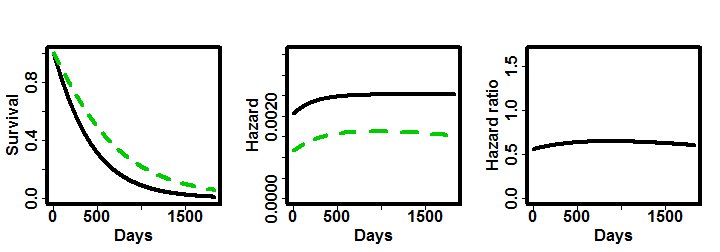Note that the hazard ratio now does not monotonically increase. Curiously, when we assume a larger prevalence of the subgroup, the HR is more flat across time and the violation of the non-proportionality is atenuated. As two different mechamisms, progression and predictive subgroup, are affecting the pattern of the HR, it may not be straightforward to know in advance whether the non-proportional hazards assumption will be met or not. The nph package is of great help to model and visualise possible patterns.

times <- c(0, 5 * 365)   # Time interval boundaries, in days

# Treatment group
t_resp <-  c(.5, .5)
B5 <- pop_pchaz(
T = times,
lambdaMat1    = m2r(matrix(c(30,
18),nrow = 2)),
lambdaMat2    = m2r(matrix(c(20,
11),nrow = 2)),
lambdaProgMat = m2r(matrix(c(15,
9), nrow = 2)),
p = t_resp,
timezero = FALSE, discrete_approximation = TRUE
)

# Control group
c_resp <- 1
K5  <- pop_pchaz(
T = times,
lambdaMat1    = m2r(matrix(11,nrow = 1)),
lambdaMat2    = m2r(matrix(9, nrow = 1)),
lambdaProgMat = m2r(matrix(5, nrow = 1)),
p = c_resp,
timezero = TRUE, discrete_approximation = TRUE
)
plot_shhr(K5, B5)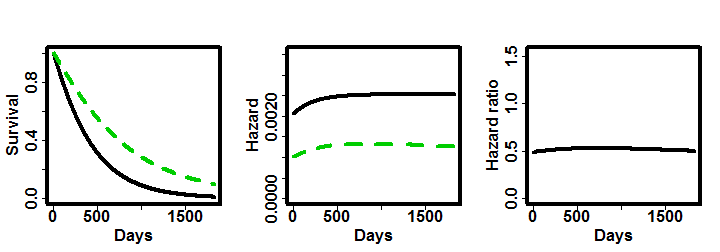Further assume that the median OS is the same before and after progression for the subgroup. This is usually reffered to as the “cure” model.

times <- c(0, 5 * 365)   # Time interval boundaries, in days

# Treatment group
t_resp <-  c(.5, .5)
B5 <- pop_pchaz(
T = times,
lambdaMat1    = m2r(matrix(c(30,
18), nrow = 2)),
lambdaMat2    = m2r(matrix(c(30,
11), nrow = 2)),
lambdaProgMat = m2r(matrix(c(15,
9), nrow = 2)),
p = t_resp,
timezero = FALSE, discrete_approximation = TRUE
)

# Control group
c_resp <- 1
K5  <- pop_pchaz(
T = times,
lambdaMat1    = m2r(matrix(11, nrow = 1)),
lambdaMat2    = m2r(matrix( 9, nrow = 1)),
lambdaProgMat = m2r(matrix( 5, nrow = 1)),
p = c_resp,
timezero = TRUE, discrete_approximation = TRUE
)

pp = plot_diagram(B5, K5, A_subgr_labels = c("S1", "S2"))
pp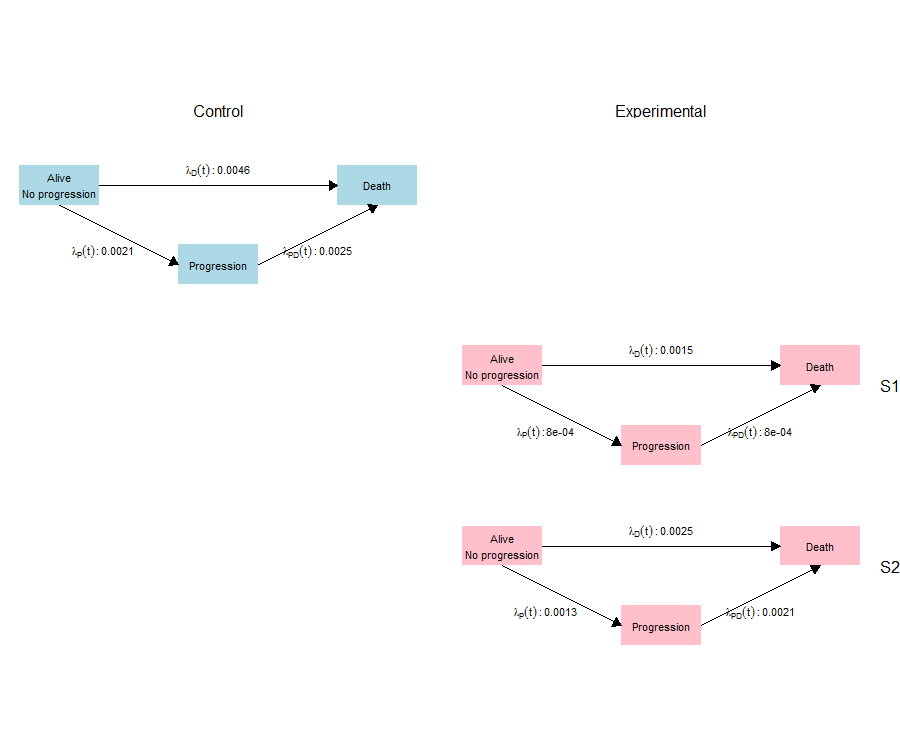plot_shhr(K5, B5)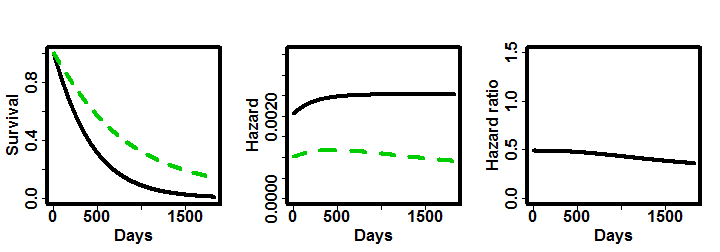Delayed Effect

Another mechamisnm that affects the hazard ratio across time is a delayed drug effect. For instance, assume that in the first 100 days of treatment, the experimental and control treatments have the same median survival time. After day 100 ($$t>100$$), the experimental treatment has a longer OS (median OS 18 and 11 before and after progression, respectively).

times <- c(0, 100, 5 * 365)   # Time interval boundaries, in days

# Treatment group
t_resp <- 1               # There are no subgroups
B5 <- pop_pchaz(
T = times,
lambdaMat1    = m2r(matrix(c(11, 18), nrow = 1)),
lambdaMat2    = m2r(matrix(c( 9, 11), nrow = 1)),
lambdaProgMat = m2r(matrix(c( 5,  9), nrow = 1)),
p = t_resp,
timezero = FALSE, discrete_approximation = TRUE
)

# Control group
c_resp <- 1
K5  <- pop_pchaz(
T = times,
lambdaMat1    = m2r(matrix(c(11, 11), nrow = 1)),
lambdaMat2    = m2r(matrix(c( 9,  9), nrow = 1)),
lambdaProgMat = m2r(matrix(c( 5,  5), nrow = 1)),
p = c_resp,
timezero = TRUE, discrete_approximation = TRUE
)

pp = plot_diagram(B5, K5)
pp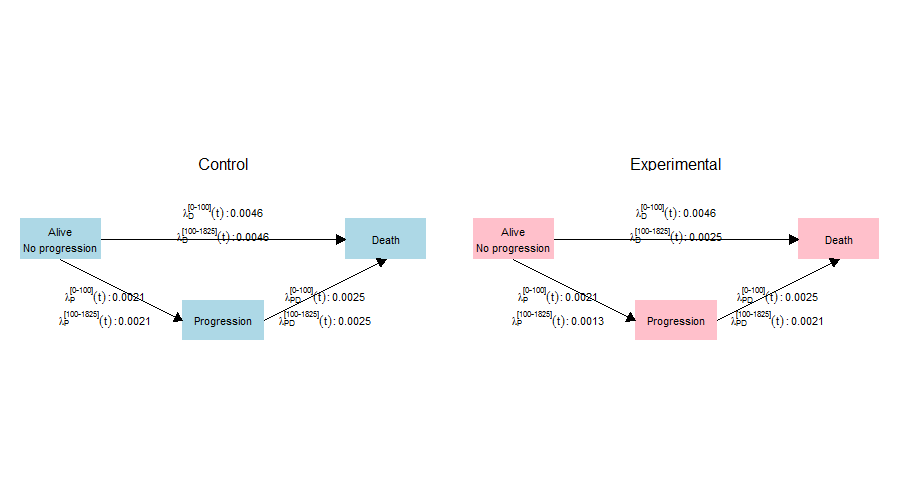plot_shhr(K5, B5)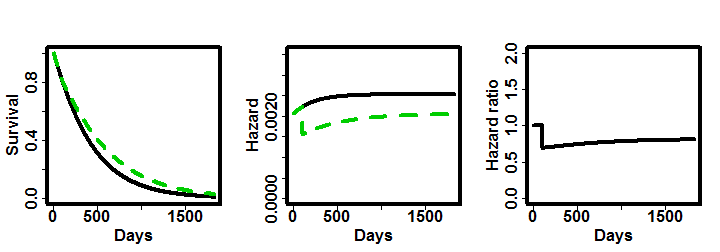Delayed Effect and Subgroup with differential effect

It is also possible to combine a delayed effect and a predictive subgroup. In this case, from days 0 to 100, the median survival times are the same for the control and experimental treatments and in both subgroups. As before, the drug starts providing a benefit after 100 days, but the subgroup has an additional benefit.

times <- c(0, 100, 5 * 365)   # Time interval boundaries, in days

# Treatment group
t_resp <- c(0.5, 0.5) #Proportion of subgroups
B5 <- pop_pchaz(
T = times,
lambdaMat1    = m2r(matrix(c(11, 30,
11, 18), byrow = TRUE, nrow = 2)),
lambdaMat2    = m2r(matrix(c( 9, 20,
9, 11), byrow = TRUE, nrow = 2)),
lambdaProgMat = m2r(matrix(c( 5, 15,
5,  9), byrow = TRUE, nrow = 2)),
p = t_resp,
timezero = FALSE, discrete_approximation = TRUE
)

# Control group
c_resp <- 1
K5  <- pop_pchaz(
T = times,
lambdaMat1    = m2r(matrix(c(11, 11), nrow = 1, ncol = 2)),
lambdaMat2    = m2r(matrix(c( 9,  9), nrow = 1, ncol = 2)),
lambdaProgMat = m2r(matrix(c( 5,  5), nrow = 1, ncol = 2)),
p = c_resp,
timezero = TRUE, discrete_approximation = TRUE
)

pp = plot_diagram(B5, K5, A_subgr_labels = c("S1", "S2"))
pp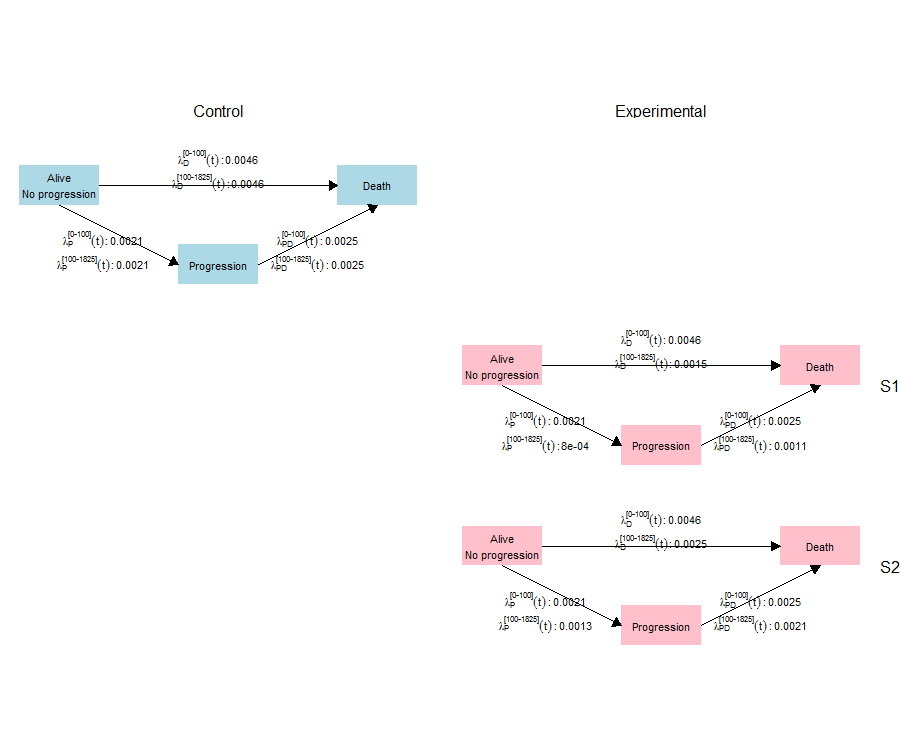plot_shhr(K5, B5)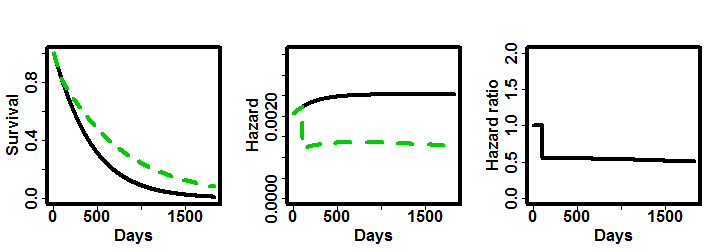Treatment Switchers

The last mechanism we explore for non-proportionality is when there are treatment switchers. In some clinical trials, patients under the control treatment are provided with the experimental treatment after their disease progresses. However, in the analysis phase, these patients will still be considered in the control group according to the intention-to-treat principle.

Assume then that after progression, 2/3 of the patients in the control treatment switch to the experimental one and therefore they have the same median survival time as in the treatment group after progression.

times <- c(0, 5 * 365)   # Time interval boundaries, in days

# Treatment group
t_resp <- 1               # There are no subgroups
B5 <- pop_pchaz(
T = times,
lambdaMat1    = m2r(matrix(18, nrow = 1)),
lambdaMat2    = m2r(matrix(11, nrow = 1)),
lambdaProgMat = m2r(matrix( 9, nrow = 1)),
p = t_resp,
timezero = FALSE, discrete_approximation = TRUE
)

# Control group
c_resp <- c(1/3, 2/3) #non-switcher, switcher reponse
K5  <- pop_pchaz(
T = times,
lambdaMat1    = m2r(matrix(c(11,
11), nrow = 2, ncol = 1)),
lambdaMat2    = m2r(matrix(c( 9,
11), nrow = 2, ncol = 1)),
lambdaProgMat = m2r(matrix(c( 5,
5), nrow = 2, ncol = 1)),
p = c_resp,
timezero = TRUE, discrete_approximation = TRUE
)

pp = plot_diagram(B5, K5, B_subgr_labels = c("Non-switcher","Switcher"))

pp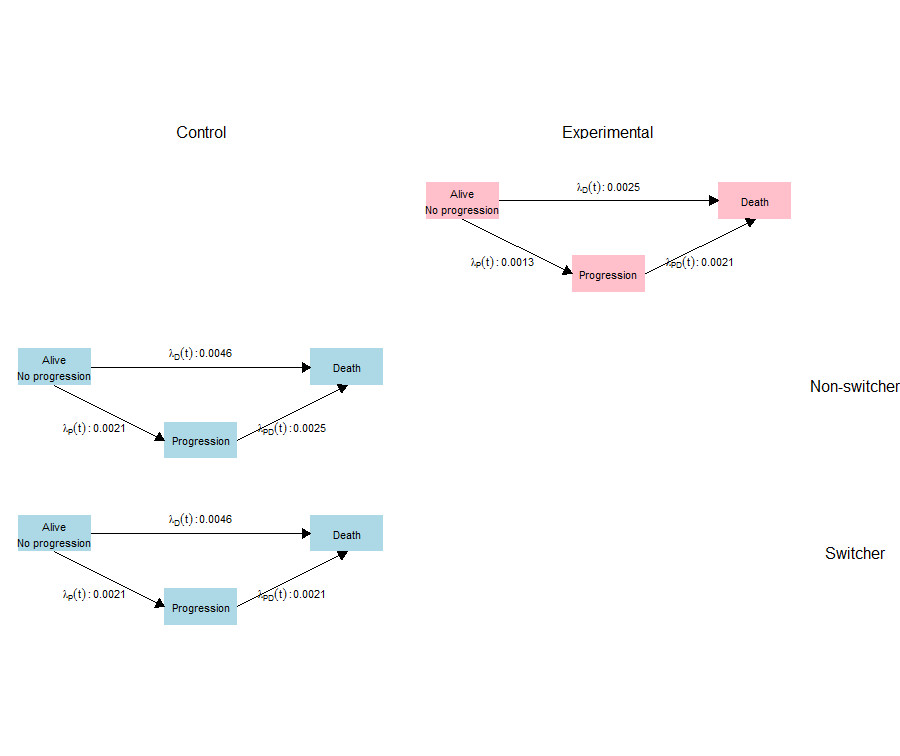plot_shhr(K5, B5)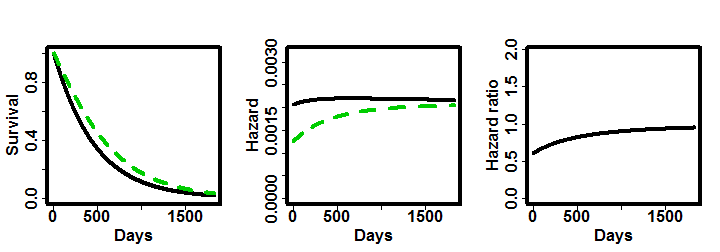It would also be sensible to assume that switchers have a higher median survival, but slightly lower median survival time when compared to the treatment group before progression.

times <- c(0, 5 * 365)   # Time interval boundaries, in days

# Treatment group
t_resp <- 1               # There are no subgroups
B5 <- pop_pchaz(
T = times,
lambdaMat1    = m2r(matrix(18, nrow = 1)),
lambdaMat2    = m2r(matrix(11, nrow = 1)),
lambdaProgMat = m2r(matrix( 9, nrow = 1)),
p = t_resp,
timezero = FALSE, discrete_approximation = TRUE
)

# Control group
c_resp <- c(1/3, 2/3) #non-switcher, switcher reponse
K5  <- pop_pchaz(
T = times,
lambdaMat1    = m2r(matrix(c(11,
11), nrow = 2, ncol = 1)),
lambdaMat2    = m2r(matrix(c( 9,
14), nrow = 2, ncol = 1)),
lambdaProgMat = m2r(matrix(c( 5,
5), nrow = 2, ncol = 1)),
p = c_resp,
timezero = TRUE, discrete_approximation = TRUE
)

pp = plot_diagram(B5, K5, B_subgr_labels = c("Non-switcher","Switcher"))
pp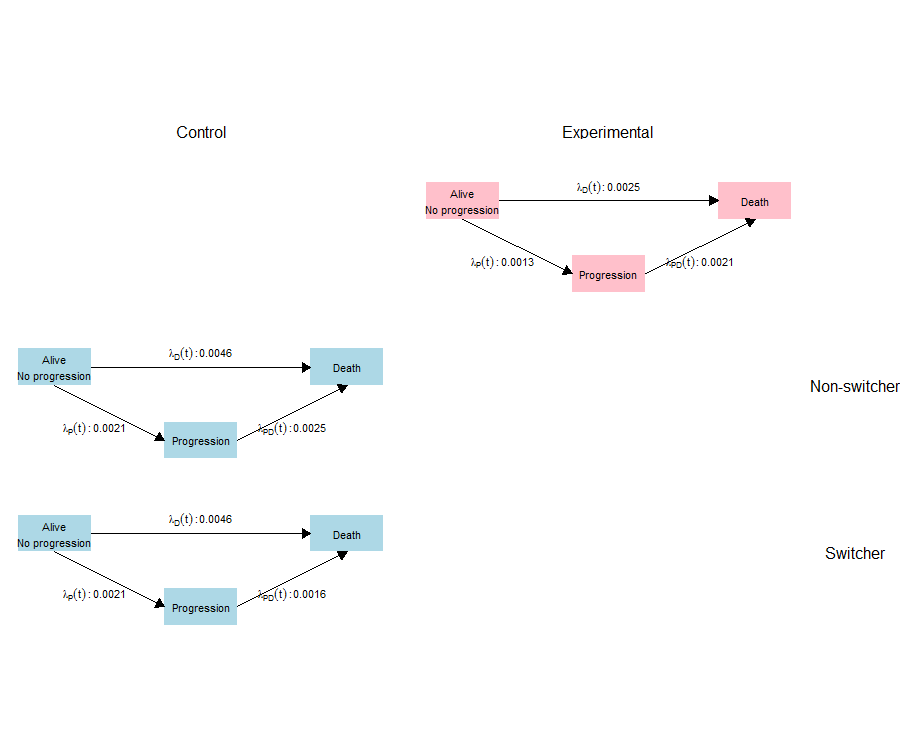plot_shhr(K5, B5)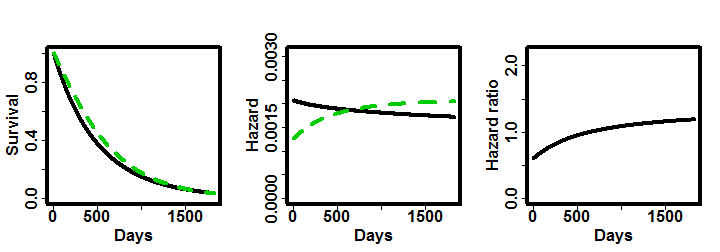Different proportion of treatment switchers may have a different effect on the hazard ratio. For example, the following two cases are identical to the two previous ones, but we change the proportion of treatment switcher to 1/3 instead of 2/3.

times <- c(0, 5 * 365)   # Time interval boundaries, in days

# Treatment group
t_resp <- 1               # There are no subgroups
B5 <- pop_pchaz(
T = times,
lambdaMat1    = m2r(matrix(18,nrow = 1)),
lambdaMat2    = m2r(matrix(11,nrow = 1)),
lambdaProgMat = m2r(matrix(9, nrow = 1)),
p = t_resp,
timezero = FALSE, discrete_approximation = TRUE
)

# Control group
c_resp <- c(2/3, 1/3) #non-switcher, switcher reponse
K5  <- pop_pchaz(
T = times,
lambdaMat1    = m2r(matrix(c(11,
11), nrow = 2, ncol = 1)),
lambdaMat2    = m2r(matrix(c(9,
11), nrow = 2, ncol = 1)),
lambdaProgMat = m2r(matrix(c(5,
5),  nrow = 2, ncol = 1)),
p = c_resp,
timezero = TRUE, discrete_approximation = TRUE
)

pp = plot_diagram(B5, K5, B_subgr_labels = c("Non-switcher","Switcher"))
ppplot_shhr(K5, B5)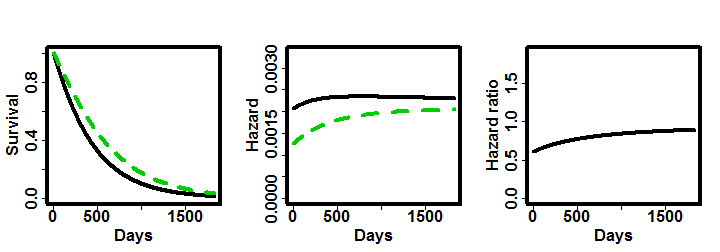times <- c(0, 5 * 365)   # Time interval boundaries, in days

# Treatment group
t_resp <- 1               # There are no subgroups
B5 <- pop_pchaz(
T = times,
lambdaMat1    = m2r(matrix(18, nrow = 1)),
lambdaMat2    = m2r(matrix(11, nrow = 1)),
lambdaProgMat = m2r(matrix( 9, nrow = 1)),
p = t_resp,
timezero = FALSE, discrete_approximation = TRUE
)

# Control group
c_resp <- c(2/3, 1/3) #non-switcher, switcher reponse
K5  <- pop_pchaz(
T = times,
lambdaMat1    = m2r(matrix(c(11,
11), nrow = 2, ncol = 1)),
lambdaMat2    = m2r(matrix(c( 9,
14), nrow = 2, ncol = 1)),
lambdaProgMat = m2r(matrix(c( 5,
5), nrow = 2, ncol = 1)),
p = c_resp,
timezero = TRUE, discrete_approximation = TRUE
)

pp = plot_diagram(B5, K5, B_subgr_labels = c("Non-switcher","Switcher"))
ppplot_shhr(K5, B5)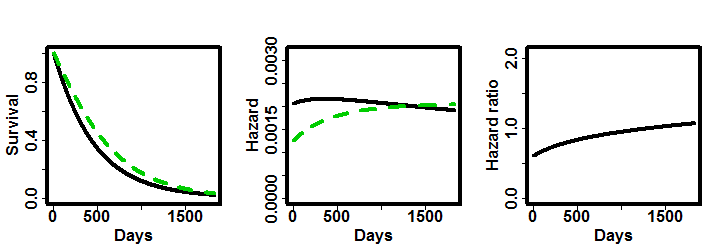Harmful drug in a subgroup but beneficial in the complement.

Some interesting scenarios may arise. For example, consider of a drug that harms a subgroup decreasing their survival time, but providing benefit to the complement of the subgroup. This scenario results in crossing survival curves, which leads to a decreasing hazard ratio.

times <- c(0, 5 * 365)   # Time interval boundaries, in days

# Treatment group
t_resp <- c(.5, .5)
B5 <- pop_pchaz(
T = times,
lambdaMat1    = m2r(matrix(c( 2,
18), nrow = 2)),
lambdaMat2    = m2r(matrix(c( 2,
18), nrow = 2)),
lambdaProgMat = m2r(matrix(c(19,
19), nrow = 2)),
p = t_resp,
timezero = FALSE, discrete_approximation = TRUE
)

# Control group
c_resp <- c(1)
K5  <- pop_pchaz(
T = times,
lambdaMat1    = m2r(matrix(c(11), nrow = 1, ncol = 1)),
lambdaMat2    = m2r(matrix(c( 9), nrow = 1, ncol = 1)),
lambdaProgMat = m2r(matrix(c( 5), nrow = 1, ncol = 1)),
p = c_resp,
timezero = TRUE, discrete_approximation = TRUE
)

pp = plot_diagram(B5, K5,
A_subgr_labels = c("S1", "S2"))
pp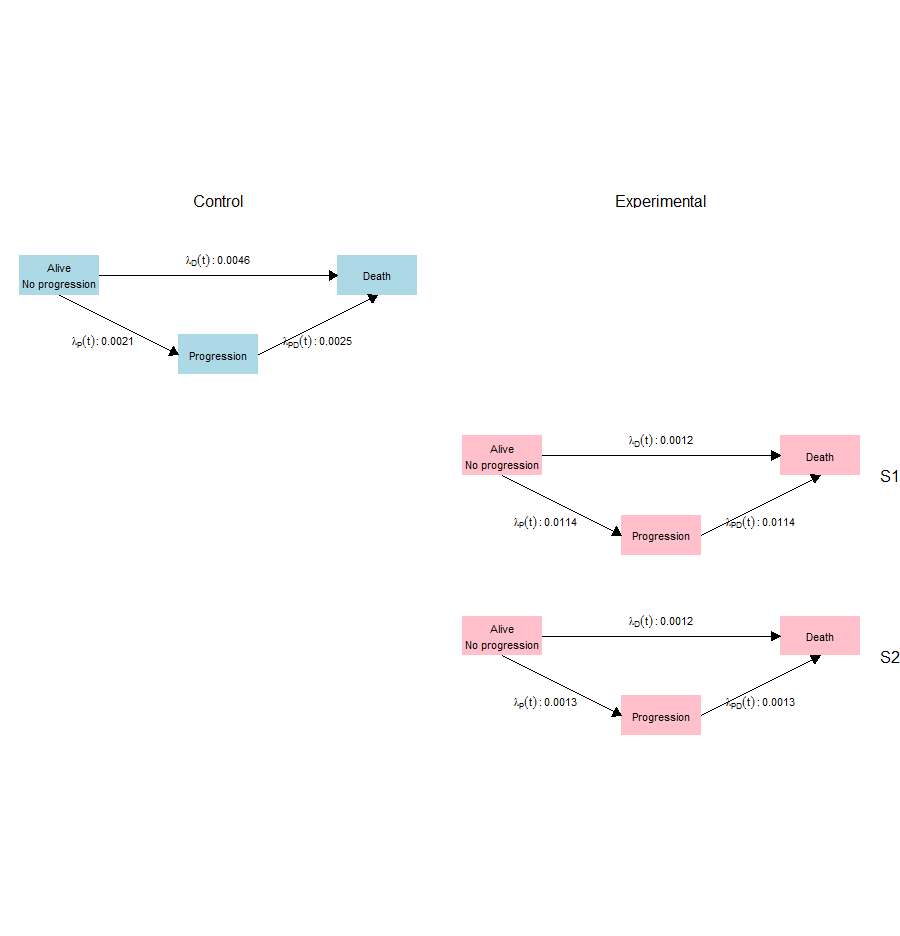plot_shhr(K5, B5)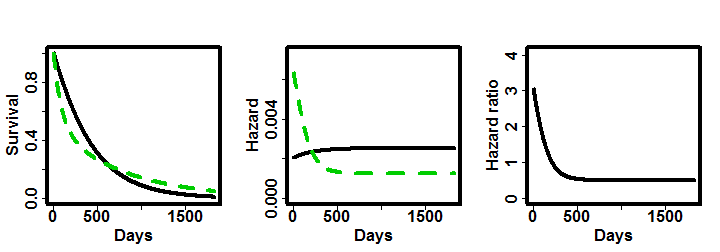Switchers and subgroup with differential effect.

We now combine the case of treatment switchers with the case of a predictive subgroup. In this case, we also address the issue that the treatment switchers may also respond differently to the drug according to whether they belong to the predictive subgroup.

In the following cases we add the predictive subgroups with treatment switchers using different median survival and switchers proportions.

First, lets examine the cure model.

times <- c(0, 5 * 365)   # Time interval boundaries, in days

# Treatment group
t_resp <- c(.2, .8)
B5 <- pop_pchaz(
T = times,
lambdaMat1    = m2r(matrix(c(30,
18), nrow = 2)),
lambdaMat2    = m2r(matrix(c(30,
11), nrow = 2)),
lambdaProgMat = m2r(matrix(c(15,
9), nrow = 2)),
p = t_resp,
timezero = FALSE, discrete_approximation = TRUE
)

# Control group
c_resp <- c(2/3, 1/3*t_resp, 1/3*t_resp)
K5  <- pop_pchaz(
T = times,
lambdaMat1    = m2r(matrix(c(11), nrow = 3, ncol = 1)),
lambdaMat2    = m2r(matrix(c( 9,
25,
14), nrow = 3)),
lambdaProgMat = m2r(matrix(c( 5), nrow = 3,ncol = 1)),
p = c_resp,
timezero = TRUE, discrete_approximation = TRUE
)

pp = plot_diagram(B5, K5,
A_subgr_labels = c("S1", "S2"),
B_subgr_labels = c("Non-switcher","Switcher-S1","Switcher-S2"))
pp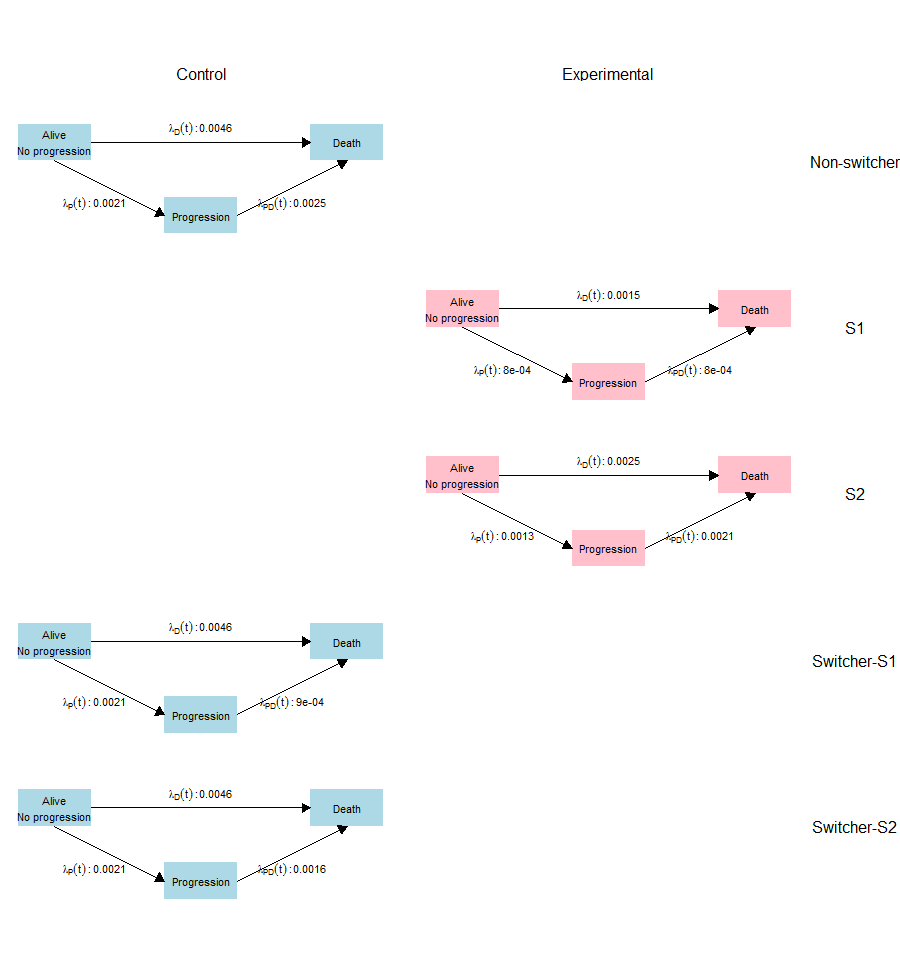plot_shhr(K5, B5)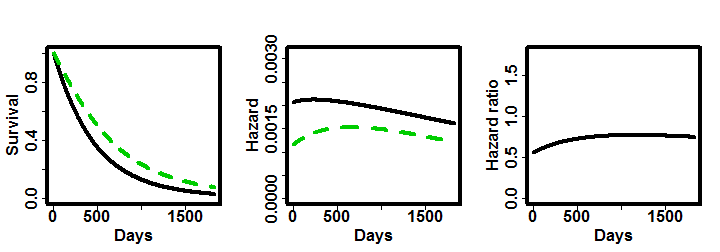Now subgroup prevalence is 0.5.

times <- c(0, 5 * 365)   # Time interval boundaries, in days

# Treatment group
t_resp <- c(.5, .5)
B5 <- pop_pchaz(
T = times,
lambdaMat1    = m2r(matrix(c(30,
18), nrow = 2)),
lambdaMat2    = m2r(matrix(c(30,
11), nrow = 2)),
lambdaProgMat = m2r(matrix(c(15,
9), nrow = 2)),
p = t_resp,
timezero = FALSE, discrete_approximation = TRUE
)

# Control group
c_resp <- c(2/3, 1/3*t_resp, 1/3*t_resp)
K5  <- pop_pchaz(
T = times,
lambdaMat1    = m2r(matrix(c(11), nrow = 3, ncol = 1)),
lambdaMat2    = m2r(matrix(c( 9,
25,
14), nrow = 3)),
lambdaProgMat = m2r(matrix(c( 5), nrow = 3,ncol = 1)),
p = c_resp,
timezero = TRUE, discrete_approximation = TRUE
)

pp = plot_diagram(B5, K5,
A_subgr_labels = c("S1", "S2"),
B_subgr_labels = c("Non-switcher","Switcher-S1","Switcher-S2"))
ppplot_shhr(K5, B5)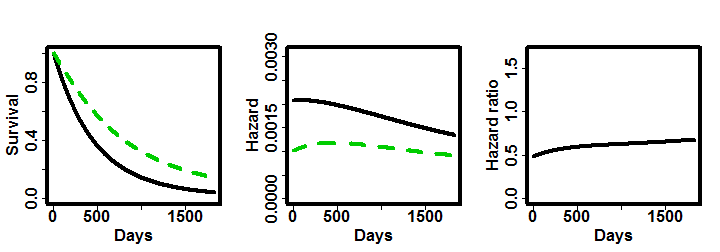Now proportion of switchers is 2/3.

times <- c(0, 5 * 365)   # Time interval boundaries, in days

# Treatment group
t_resp <- c(.5, .5)
B5 <- pop_pchaz(
T = times,
lambdaMat1    = m2r(matrix(c(30,
18), nrow = 2)),
lambdaMat2    = m2r(matrix(c(20,
11), nrow = 2)),
lambdaProgMat = m2r(matrix(c(15,
9), nrow = 2)),
p = t_resp,
timezero = FALSE, discrete_approximation = TRUE
)

# Control group
c_resp <- c(1/3, 2/3*t_resp, 2/3*t_resp)
K5  <- pop_pchaz(
T = times,
lambdaMat1    = m2r(matrix(c(11), nrow = 3, ncol = 1)),
lambdaMat2    = m2r(matrix(c( 9,
25,
14), nrow = 3)),
lambdaProgMat = m2r(matrix(c( 5), nrow = 3,ncol = 1)),
p = c_resp,
timezero = TRUE, discrete_approximation = TRUE
)

pp = plot_diagram(B5, K5,
A_subgr_labels = c("S1", "S2"),
B_subgr_labels = c("Non-switcher","Switcher-S1","Switcher-S2"))
pp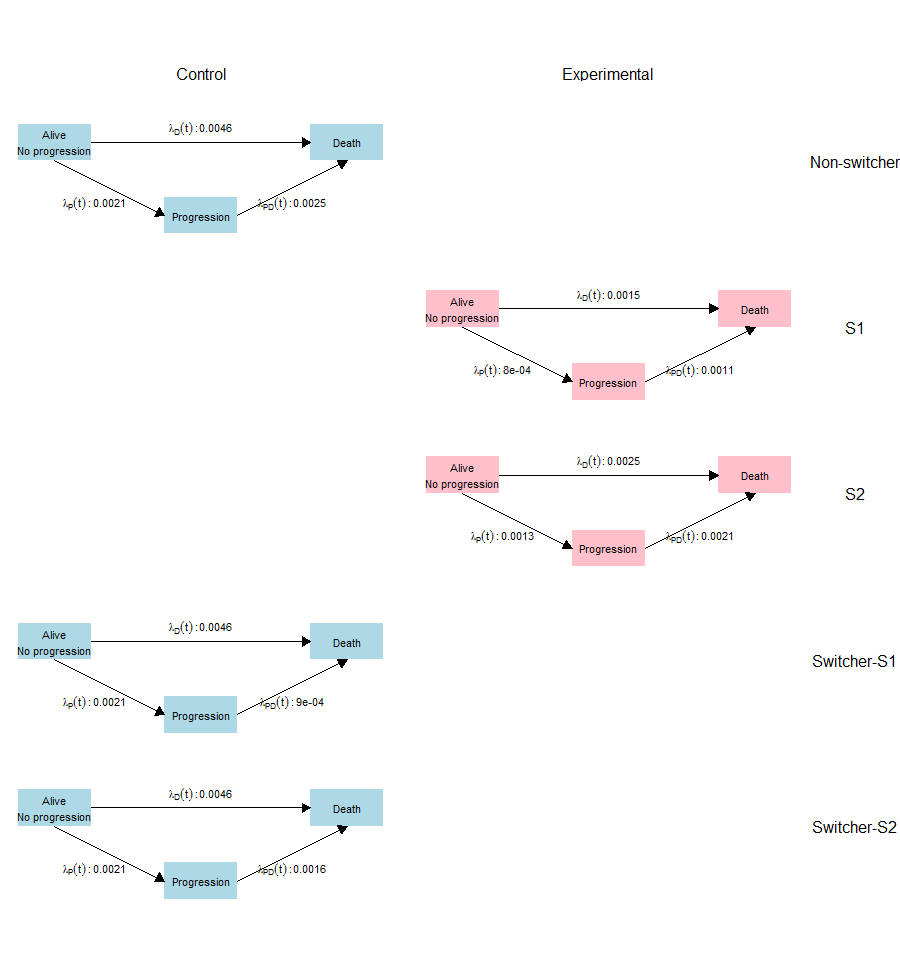plot_shhr(K5, B5)